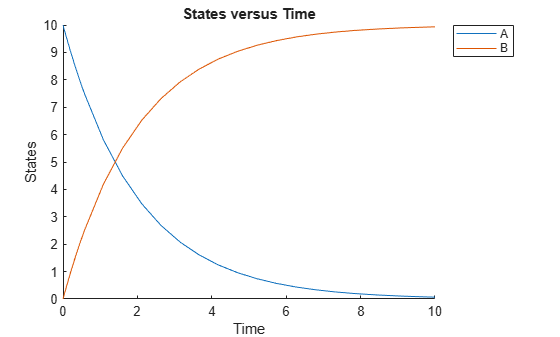# Construct a Simple Model

This example shows you how to construct a simple model with two species (A and B) and a reaction. The reaction is `A -> B`, which follows mass action kinetics with the forward rate parameter `k`. Hence the rate of change is $dA/dt=-k*A$.

Create a SimBiology model named `simpleModel`.

`m1 = sbiomodel('simpleModel');`

Add a reaction that involves two species `A` and `B`, where `A` is converted to `B`.

`r1 = addreaction(m1,'A -> B');`

SimBiology automatically add species `A` and `B` to the model.

`m1.species`
```ans = SimBiology Species Array Index: Compartment: Name: Value: Units: 1 unnamed A 0 2 unnamed B 0 ```

Set the initial amount of the first species (`A`) to 10.

`m1.species(1).InitialAmount = 10;`

Define the kinetic law of the reaction to follow mass action kinetics. You can achieve this by adding a kinetic law object to the reaction `r1`.

`kineticLaw = addkineticlaw(r1,'MassAction');`

Add a rate constant parameter to the mass action kinetic law. You must set the `ParameterVariableNames` property of the kinetic law object to the name of the parameter `'k'` so that the reaction rate can be determined.

```p1 = addparameter(kineticLaw,'k',0.5); kineticLaw.ParameterVariableNames = 'k';```

Simulate the model.

`sd = sbiosimulate(m1);`

Plot the simulation results.

`sbioplot(sd);`